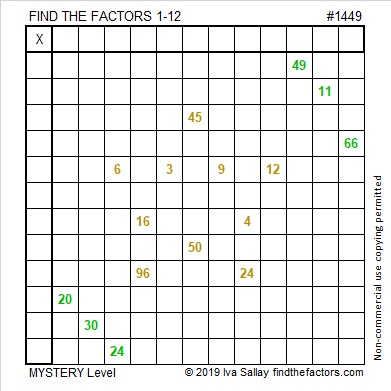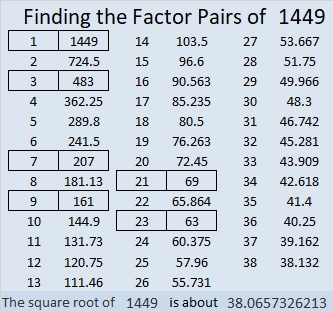# 1449 Christmas Star

If you’ve ever wished you knew the multiplication table better, then make that wish upon this Christmas star. If you use logic and don’t give up,  then you can watch your wish come true!I number the puzzles to distinguish them from one another. That star puzzle is way too big for a factor tree made with its puzzle number:Here’s more about the number 1449:

• Prime factorization: 1449 = 3 × 3 × 7 × 23, which can be written 1449 = 3² × 7 × 23
• 1449 has at least one exponent greater than 1 in its prime factorization so √1449 can be simplified. Taking the factor pair from the factor pair table below with the largest square number factor, we get √1449 = (√9)(√161) = 3√161
• The exponents in the prime factorization are 2, 1, and 1. Adding one to each exponent and multiplying we get (2 + 1)(1 + 1)(1 + 1) = 3 × 2 × 2 = 12. Therefore 1449 has exactly 12 factors.
• The factors of 1449 are outlined with their factor pair partners in the graphic below.1449 is the difference of two squares in 6 different ways:
725² – 724² = 1449
243² – 240² = 1449
107²-100² = 1449
85² – 76² = 1449
45² – 24² = 1449
43² – 20² = 1449